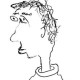# What does a general proper rigid space look like?

As the title says. Consider proper rigid spaces$X$ over some nonarchimedean field$K$. The “standard” examples of such things which don’t come from algebraic geometry are i) the Hopf surface$(\mathbf{A}^2 - 0)/p^\mathbf{Z}$, ii) non-algebraizable deformations of K3 surfaces over the residue field of$K$, and iii) generic abeloid varieties (which are analogous to generic compact complex tori).  But there must be gazillions of other examples, right? A “random” proper rigid space is hard to write down, sort of by definition. But there are certainly some natural questions one can ask:

-For every$n \geq 2$, does there exist a proper$n$-dimensional rigid space with no non-constant meromorphic functions, and admitting a formal model whose special fiber has components of general type? Can we find examples of such spaces with arbitrarily large dimension dimension which don’t come from lower-dimensional examples by simple operations (products, quotients by finite groups, etc.)? Same question but with “no non-constant meromorphic functions” replaced by the weaker requirement that$\mathrm{tr.deg}K(X)/K$ is small compared to$\dim X$.

-Do there exist non-algebraizable proper rigid spaces with “arbitrarily bad” singularities?

-Do there exist rigid analytic analogues of Kodaira’s class VII0 surfaces?

## 2 thoughts on “What does a general proper rigid space look like?”

1.mayorliatmath says:

Is it clear if the transcendence degree of K(X) over K is finite when X is proper over K?

I was wondering whether every, say, isolated singularity in rigid geometry is algebraizable. (so “arbitrarily bad” could mean even worse than algebraic ones, namely really really bad.)

Like

1.arithmetica says:

I cannot find an actual “classification” in that paper!

Yes, it’s always bounded above by$\dim X$, see Conrad’s article on Moishezon spaces.

I think isolated singularities can be algebraized, cf. Theorem 3.8 in (http://www.numdam.org/article/PMIHES_1969__36__23_0.pdf).

Like